SLVSDN2E January   2018  – March 2021

PRODUCTION DATA

1. Features
2. Applications
3. Description
4. Revision History
5. Pin Configuration and Functions
6. Specifications
7. Detailed Description
1. 7.1 Overview
2. 7.2 Functional Block Diagram
3. 7.3 Feature Description
4. 7.4 Device Functional Modes
8. Application and Implementation
1. 8.1 Application Information
2. 8.2 Typical Application
9. Power Supply Recommendations
10. 10Layout
11. 11Device and Documentation Support
12. 12Mechanical, Packaging, and Orderable Information

• RUM|16
• PWP|16
• RUM|16
• PWP|16

### 7.3.1 Speed Input and Control

The DRV10974 device has a three-phase 25-kHz PWM (fPWM_OUT) output that has an average value of sinusoidal waveforms from phase to phase as shown in Figure 7-2. When any phase is measured with reference to ground, the waveform observed is a PWM-encoded sinusoid coupled with third-order harmonics as shown in Figure 7-3. This encoding scheme simplifies the driver requirements because one phase output is always equal to zero.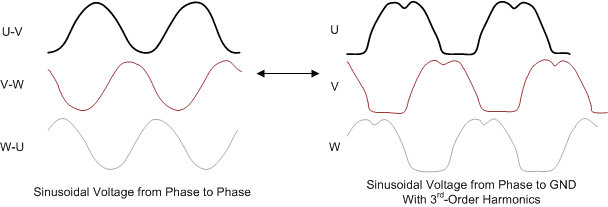Figure 7-2 Sinusoidal VoltageFigure 7-3 PWM Encoded Phase Output and the Average Value

The output amplitude is determined by the supply voltage (VCC) and the PWM-commanded duty cycle (PWM) as calculated in Equation 1 and shown in Figure 7-4. The maximum amplitude is applied when the commanded PWM duty cycle is slightly less than 100% in order to keep the 25-kHz PWM rate (fPWM_OUT).

Equation 1.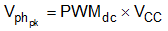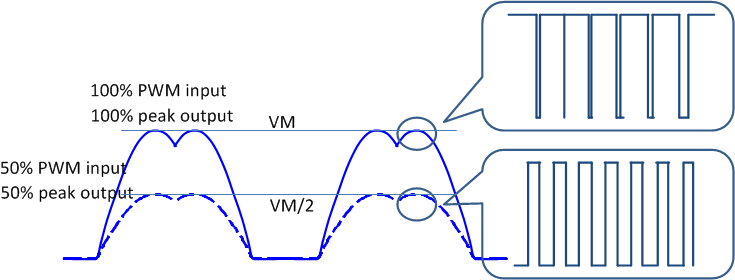Figure 7-4 Output Voltage Amplitude Adjustment

The motor speed is controlled indirectly by using the PWM command to control the amplitude of the phase voltages which are applied to the motor. The PWM pin can be driven by either a digital duty cycle or an analog voltage.

The duty cycle of the PWM input (PWM) is passed through a low-pass filter that ramps from 0% to 100% duty cycle in 120 ms. The control resolution is approximately 0.2% (DCSTEP). The signal path from PWM input to PWM motor is shown in Figure 7-5.Figure 7-5 PWM Command Input Control Diagram

The output peak amplitude is described by Equation 1 when PWMdc > 15% (the minimum-operation duty cycle). When the PWM-commanded duty cycle is lower than the minimum-operation duty cycle and higher than 1.5% (DCON_MIN), the output is controlled the by the minimum-operation duty cycle (DCMIN). This is shown in Figure 7-6 for analog input, and for duty cycles greater than 1.5% (DCON_MIN) for digital input. If the supply voltage (VVCC) > 14 V, the maximum PWMdc is limited to 14 V / VVCC.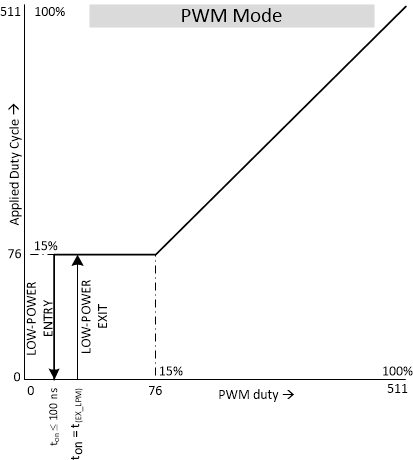Figure 7-6 PWM-Mode Speed-Control Transfer Function

When the PWM pin is driven with an analog voltage, the output peak amplitude depends on the supply voltage, the analog voltage on the PWM pin (VANA), and the voltage of V1P8 (VV1P8). This is shown in Equation 2:

Equation 2.Note the output peak amplitude is described by Equation 2 when the VANA > 0.27 V or 15% of 1.8 V. This is the equivalent of the minimum-operation duty cycle percentage of 15% (DCMIN). When the analog voltage on the PWM pin is lower than the minimum-operation duty-cycle percentage but higher than the zero-speed analog voltage (VANA_ZS), the output is controlled by the minimum-operation duty cycle. When the analog voltage on the PWM pin is below zero-speed analog voltage, the DRV10974 enters low-power mode. This is shown in Figure 7-7.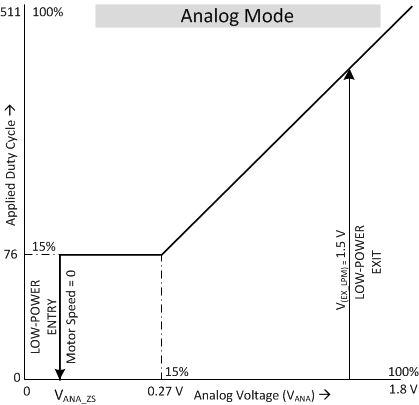Figure 7-7 Analog-Mode Speed-Control Transfer Function